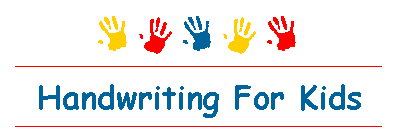Web www.handwritingforkids.com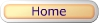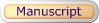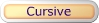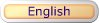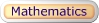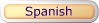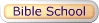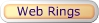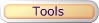### Mathematics - Addition

Animated Math Teacher

### How to Add: 1 + 2 = 3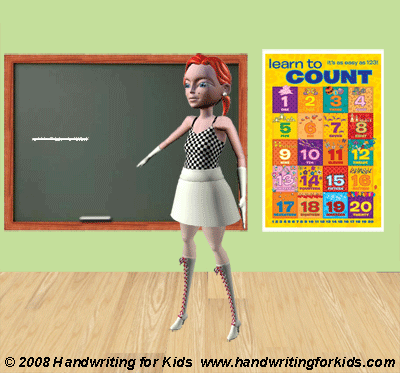The numbers that are added together in a math addition problem are called ADDENDS.

The answer in a addition math problem is called the SUM.

 1 + 2 = 3 addend addend sum

You can also draw a picture or dots to find a sum.

 1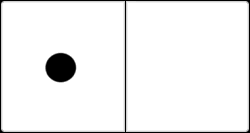+ 2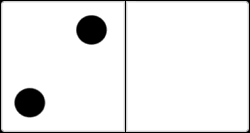= 3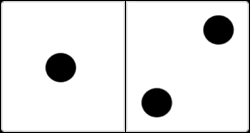1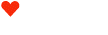+ 2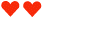= 3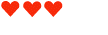You can find more addition math worksheets located at:

or

 Level 1: Visual Math - ALL NEW Exercises! Hearts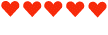DominoesPlease let us know how you like the above animation and instructions. Would you like to see more of this type of instructions at our website? Drop us an email and give us feedback would be appreciated. Thank you!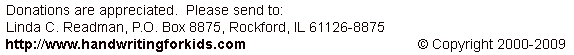Basic Handwriting for Kids Free for Non-Profit Use Ms. Readman, Rockford, IL Revised: